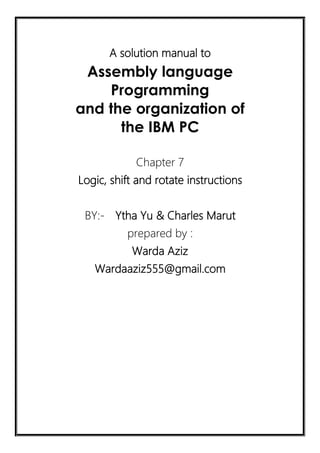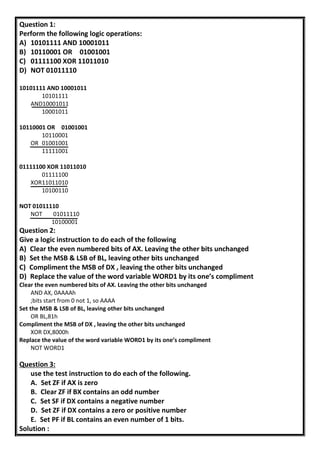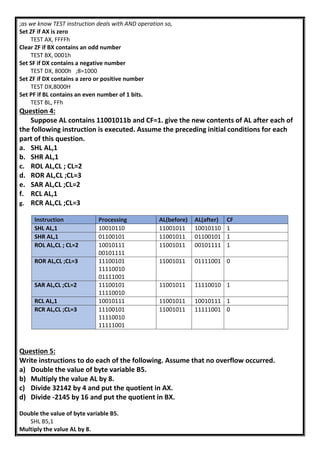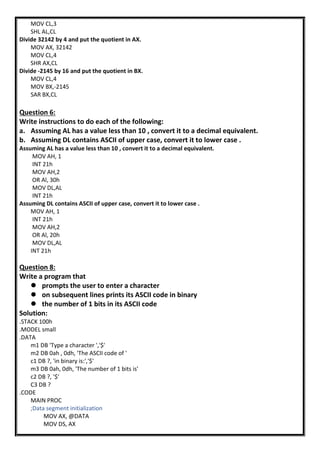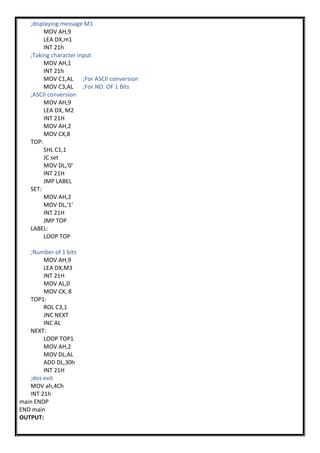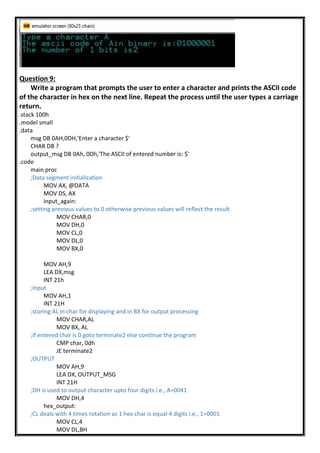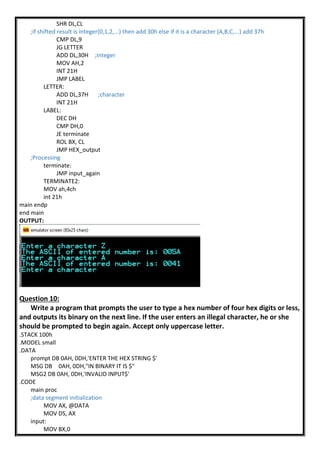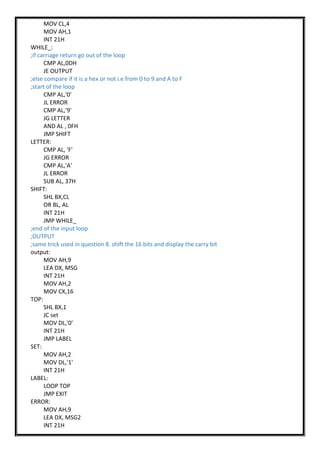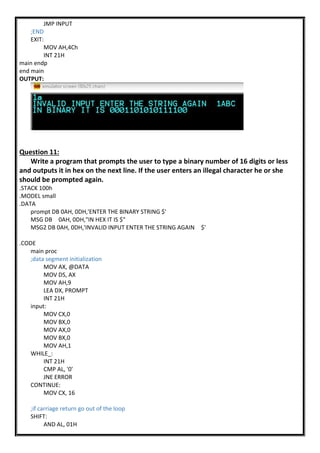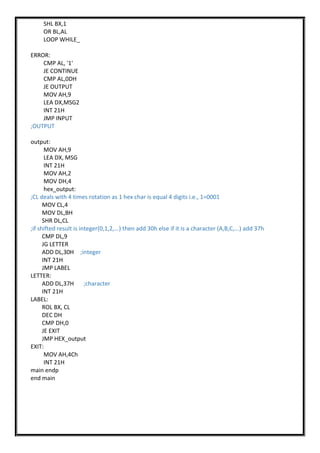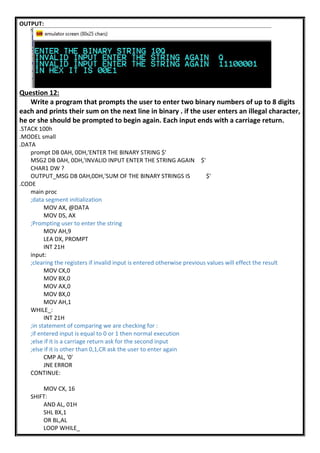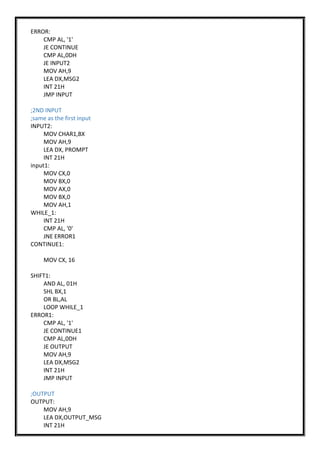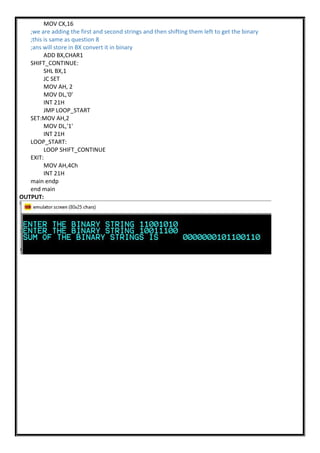Próximo SlideShare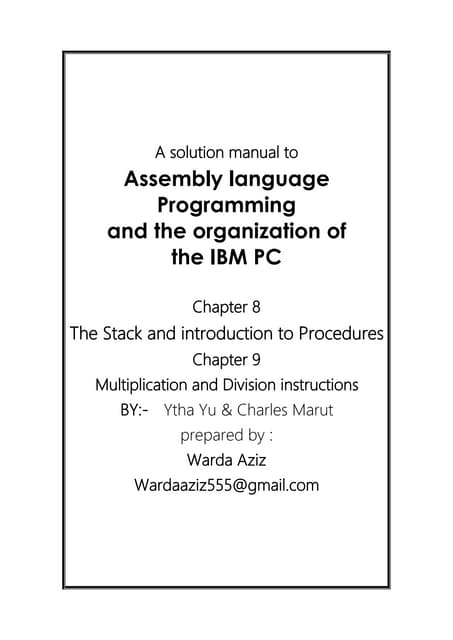Chap 8 The stack and introduction to procedures & Chapter 9 multiplication an...
Cargando en ... 3
1 de 13

### chapter 7 Logic, shift and rotate instructions

1. A solution manual to Assembly language Programming and the organization of the IBM PC Chapter 7 Logic, shift and rotate instructions BY:- Ytha Yu & Charles Marut prepared by : Warda Aziz Wardaaziz555@gmail.com
2. Question 1: Perform the following logic operations: A) 10101111 AND 10001011 B) 10110001 OR 01001001 C) 01111100 XOR 11011010 D) NOT 01011110 10101111 AND 10001011 10101111 AND10001011 10001011 10110001 OR 01001001 10110001 OR 01001001 11111001 01111100 XOR 11011010 01111100 XOR11011010 10100110 NOT 01011110 NOT 01011110 10100001 Question 2: Give a logic instruction to do each of the following A) Clear the even numbered bits of AX. Leaving the other bits unchanged B) Set the MSB & LSB of BL, leaving other bits unchanged C) Compliment the MSB of DX , leaving the other bits unchanged D) Replace the value of the word variable WORD1 by its one’s compliment Clear the even numbered bits of AX. Leaving the other bits unchanged AND AX, 0AAAAh ;bits start from 0 not 1, so AAAA Set the MSB & LSB of BL, leaving other bits unchanged OR BL,81h Compliment the MSB of DX , leaving the other bits unchanged XOR DX,8000h Replace the value of the word variable WORD1 by its one’s compliment NOT WORD1 Question 3: use the test instruction to do each of the following. A. Set ZF if AX is zero B. Clear ZF if BX contains an odd number C. Set SF if DX contains a negative number D. Set ZF if DX contains a zero or positive number E. Set PF if BL contains an even number of 1 bits. Solution :
3. ;as we know TEST instruction deals with AND operation so, Set ZF if AX is zero TEST AX, FFFFh Clear ZF if BX contains an odd number TEST BX, 0001h Set SF if DX contains a negative number TEST DX, 8000h ;8=1000 Set ZF if DX contains a zero or positive number TEST DX,8000H Set PF if BL contains an even number of 1 bits. TEST BL, FFh Question 4: Suppose AL contains 11001011b and CF=1. give the new contents of AL after each of the following instruction is executed. Assume the preceding initial conditions for each part of this question. a. SHL AL,1 b. SHR AL,1 c. ROL AL,CL ; CL=2 d. ROR AL,CL ;CL=3 e. SAR AL,CL ;CL=2 f. RCL AL,1 g. RCR AL,CL ;CL=3 Question 5: Write instructions to do each of the following. Assume that no overflow occurred. a) Double the value of byte variable B5. b) Multiply the value AL by 8. c) Divide 32142 by 4 and put the quotient in AX. d) Divide -2145 by 16 and put the quotient in BX. Double the value of byte variable B5. SHL B5,1 Multiply the value AL by 8. Instruction Processing AL(before) AL(after) CF SHL AL,1 10010110 11001011 10010110 1 SHR AL,1 01100101 11001011 01100101 1 ROL AL,CL ; CL=2 10010111 00101111 11001011 00101111 1 ROR AL,CL ;CL=3 11100101 11110010 01111001 11001011 01111001 0 SAR AL,CL ;CL=2 11100101 11110010 11001011 11110010 1 RCL AL,1 10010111 11001011 10010111 1 RCR AL,CL ;CL=3 11100101 11110010 11111001 11001011 11111001 0
4. MOV CL,3 SHL AL,CL Divide 32142 by 4 and put the quotient in AX. MOV AX, 32142 MOV CL,4 SHR AX,CL Divide -2145 by 16 and put the quotient in BX. MOV CL,4 MOV BX,-2145 SAR BX,CL Question 6: Write instructions to do each of the following: a. Assuming AL has a value less than 10 , convert it to a decimal equivalent. b. Assuming DL contains ASCII of upper case, convert it to lower case . Assuming AL has a value less than 10 , convert it to a decimal equivalent. MOV AH, 1 INT 21h MOV AH,2 OR Al, 30h MOV DL,AL INT 21h Assuming DL contains ASCII of upper case, convert it to lower case . MOV AH, 1 INT 21h MOV AH,2 OR Al, 20h MOV DL,AL INT 21h Question 8: Write a program that  prompts the user to enter a character  on subsequent lines prints its ASCII code in binary  the number of 1 bits in its ASCII code Solution: .STACK 100h .MODEL small .DATA m1 DB 'Type a character ','\$' m2 DB 0ah , 0dh, 'The ASCII code of ' c1 DB ?, 'in binary is:','\$' m3 DB 0ah, 0dh, 'The number of 1 bits is' c2 DB ?, '\$' C3 DB ? .CODE MAIN PROC ;Data segment initialization MOV AX, @DATA MOV DS, AX
5. ;displaying message M1 MOV AH,9 LEA DX,m1 INT 21h ;Taking character input MOV AH,1 INT 21h MOV C1,AL ;For ASCII conversion MOV C3,AL ;For NO. OF 1 Bits ;ASCII conversion MOV AH,9 LEA DX, M2 INT 21H MOV AH,2 MOV CX,8 TOP: SHL C1,1 JC set MOV DL,'0' INT 21H JMP LABEL SET: MOV AH,2 MOV DL,'1' INT 21H JMP TOP LABEL: LOOP TOP ;Number of 1 bits MOV AH,9 LEA DX,M3 INT 21H MOV AL,0 MOV CX, 8 TOP1: ROL C3,1 JNC NEXT INC AL NEXT: LOOP TOP1 MOV AH,2 MOV DL,AL ADD DL,30h INT 21H ;dos exit MOV ah,4Ch INT 21h main ENDP END main OUTPUT:
6. Question 9: Write a program that prompts the user to enter a character and prints the ASCII code of the character in hex on the next line. Repeat the process until the user types a carriage return. .stack 100h .model small .data msg DB 0AH,0DH,'Enter a character \$' CHAR DB ? output_msg DB 0Ah, 0Dh,'The ASCII of entered number is: \$' .code main proc ;Data segment initialization MOV AX, @DATA MOV DS, AX input_again: ;setting previous values to 0 otherwise previous values will reflect the result MOV CHAR,0 MOV DH,0 MOV CL,0 MOV DL,0 MOV BX,0 MOV AH,9 LEA DX,msg INT 21h ;input MOV AH,1 INT 21H ;storing AL in char for displaying and in BX for output processing MOV CHAR,AL MOV BX, AL ;if entered char is 0 goto terminate2 else continue the program CMP char, 0dh JE terminate2 ;OUTPUT MOV AH,9 LEA DX, OUTPUT_MSG INT 21H ;DH is used to output character upto four digits i.e., A=0041 MOV DH,4 hex_output: ;CL deals with 4 times rotation as 1 hex char is equal 4 digits i.e., 1=0001 MOV CL,4 MOV DL,BH
7. SHR DL,CL ;if shifted result is integer(0,1,2,...) then add 30h else if it is a character (A,B,C,...) add 37h CMP DL,9 JG LETTER ADD DL,30H ;integer MOV AH,2 INT 21H JMP LABEL LETTER: ADD DL,37H ;character INT 21H LABEL: DEC DH CMP DH,0 JE terminate ROL BX, CL JMP HEX_output ;Processing terminate: JMP input_again TERMINATE2: MOV ah,4ch int 21h main endp end main OUTPUT: Question 10: Write a program that prompts the user to type a hex number of four hex digits or less, and outputs its binary on the next line. If the user enters an illegal character, he or she should be prompted to begin again. Accept only uppercase letter. .STACK 100h .MODEL small .DATA prompt DB 0AH, 0DH,'ENTER THE HEX STRING \$' MSG DB 0AH, 0DH,"IN BINARY IT IS \$" MSG2 DB 0AH, 0DH,'INVALID INPUT\$' .CODE main proc ;data segment initialization MOV AX, @DATA MOV DS, AX input: MOV BX,0
8. MOV CL,4 MOV AH,1 INT 21H WHILE_: ;if carriage return go out of the loop CMP AL,0DH JE OUTPUT ;else compare if it is a hex or not i.e from 0 to 9 and A to F ;start of the loop CMP AL,'0' JL ERROR CMP AL,'9' JG LETTER AND AL , 0FH JMP SHIFT LETTER: CMP AL, 'F' JG ERROR CMP AL,'A' JL ERROR SUB AL, 37H SHIFT: SHL BX,CL OR BL, AL INT 21H JMP WHILE_ ;end of the input loop ;OUTPUT ;same trick used in question 8. shift the 16 bits and display the carry bit output: MOV AH,9 LEA DX, MSG INT 21H MOV AH,2 MOV CX,16 TOP: SHL BX,1 JC set MOV DL,'0' INT 21H JMP LABEL SET: MOV AH,2 MOV DL,'1' INT 21H LABEL: LOOP TOP JMP EXIT ERROR: MOV AH,9 LEA DX, MSG2 INT 21H
9. JMP INPUT ;END EXIT: MOV AH,4Ch INT 21H main endp end main OUTPUT: Question 11: Write a program that prompts the user to type a binary number of 16 digits or less and outputs it in hex on the next line. If the user enters an illegal character he or she should be prompted again. .STACK 100h .MODEL small .DATA prompt DB 0AH, 0DH,'ENTER THE BINARY STRING \$' MSG DB 0AH, 0DH,"IN HEX IT IS \$" MSG2 DB 0AH, 0DH,'INVALID INPUT ENTER THE STRING AGAIN \$' .CODE main proc ;data segment initialization MOV AX, @DATA MOV DS, AX MOV AH,9 LEA DX, PROMPT INT 21H input: MOV CX,0 MOV BX,0 MOV AX,0 MOV BX,0 MOV AH,1 WHILE_: INT 21H CMP AL, '0' JNE ERROR CONTINUE: MOV CX, 16 ;if carriage return go out of the loop SHIFT: AND AL, 01H
10. SHL BX,1 OR BL,AL LOOP WHILE_ ERROR: CMP AL, '1' JE CONTINUE CMP AL,0DH JE OUTPUT MOV AH,9 LEA DX,MSG2 INT 21H JMP INPUT ;OUTPUT output: MOV AH,9 LEA DX, MSG INT 21H MOV AH,2 MOV DH,4 hex_output: ;CL deals with 4 times rotation as 1 hex char is equal 4 digits i.e., 1=0001 MOV CL,4 MOV DL,BH SHR DL,CL ;if shifted result is integer(0,1,2,...) then add 30h else if it is a character (A,B,C,...) add 37h CMP DL,9 JG LETTER ADD DL,30H ;integer INT 21H JMP LABEL LETTER: ADD DL,37H ;character INT 21H LABEL: ROL BX, CL DEC DH CMP DH,0 JE EXIT JMP HEX_output EXIT: MOV AH,4Ch INT 21H main endp end main
11. OUTPUT: Question 12: Write a program that prompts the user to enter two binary numbers of up to 8 digits each and prints their sum on the next line in binary . if the user enters an illegal character, he or she should be prompted to begin again. Each input ends with a carriage return. .STACK 100h .MODEL small .DATA prompt DB 0AH, 0DH,'ENTER THE BINARY STRING \$' MSG2 DB 0AH, 0DH,'INVALID INPUT ENTER THE STRING AGAIN \$' CHAR1 DW ? OUTPUT_MSG DB 0AH,0DH,'SUM OF THE BINARY STRINGS IS \$' .CODE main proc ;data segment initialization MOV AX, @DATA MOV DS, AX ;Prompting user to enter the string MOV AH,9 LEA DX, PROMPT INT 21H input: ;clearing the registers if invalid input is entered otherwise previous values will effect the result MOV CX,0 MOV BX,0 MOV AX,0 MOV BX,0 MOV AH,1 WHILE_: INT 21H ;in statement of comparing we are checking for : ;if entered input is equal to 0 or 1 then normal execution ;else if it is a carriage return ask for the second input ;else if it is other than 0,1,CR ask the user to enter again CMP AL, '0' JNE ERROR CONTINUE: MOV CX, 16 SHIFT: AND AL, 01H SHL BX,1 OR BL,AL LOOP WHILE_
12. ERROR: CMP AL, '1' JE CONTINUE CMP AL,0DH JE INPUT2 MOV AH,9 LEA DX,MSG2 INT 21H JMP INPUT ;2ND INPUT ;same as the first input INPUT2: MOV CHAR1,BX MOV AH,9 LEA DX, PROMPT INT 21H input1: MOV CX,0 MOV BX,0 MOV AX,0 MOV BX,0 MOV AH,1 WHILE_1: INT 21H CMP AL, '0' JNE ERROR1 CONTINUE1: MOV CX, 16 SHIFT1: AND AL, 01H SHL BX,1 OR BL,AL LOOP WHILE_1 ERROR1: CMP AL, '1' JE CONTINUE1 CMP AL,0DH JE OUTPUT MOV AH,9 LEA DX,MSG2 INT 21H JMP INPUT ;OUTPUT OUTPUT: MOV AH,9 LEA DX,OUTPUT_MSG INT 21H
13. MOV CX,16 ;we are adding the first and second strings and then shifting them left to get the binary ;this is same as question 8 ;ans will store in BX convert it in binary ADD BX,CHAR1 SHIFT_CONTINUE: SHL BX,1 JC SET MOV AH, 2 MOV DL,'0' INT 21H JMP LOOP_START SET:MOV AH,2 MOV DL,'1' INT 21H LOOP_START: LOOP SHIFT_CONTINUE EXIT: MOV AH,4Ch INT 21H main endp end main OUTPUT: